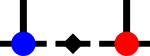SyTensyten::DenseIter< rank > Struct Template Reference

Iterator over a single dense tensor-like object. More...

#include <dense_iter.h>Collaboration diagram for syten::DenseIter< rank >:

Public Member Functions

DenseIter (std::array< Index, rank > const &dim_)
Ctor for trivial ordering (last index is increased first). More...

DenseIter (std::array< Index, rank > const &dim_, std::array< Index, rank > const &ord_)
Ctor for nontrivial ordering. More...

Size offset () const
Returns the offset from the start, mostly only valid if the ordering is trivial (but there are cases where it makes sense for non-trivial orderings as well) More...

operator bool () const
False if we reached the last element. More...

std::array< Index, rank > const & operator() () const
Returns the set of the current indices. More...

void operator++ ()
Increase the indices in the order specified by ord. More...

DenseIter operator= (DenseIter const &)=delete
Copy assignment op deleted, would require pointer fiddling. More...

DenseIter operator= (DenseIter &&)=delete
Move assignment op deleted, would require pointer fiddling. More...

Protected Attributes

bool alive
True unless we have reached the last element. More...

std::array< Index, rankcur
Current value of the array. More...

std::array< Index, rankdim
Dimensions of individual indices, dim[i] is the dimension of the i-th increased index. More...

std::array< Index *, rankptrs
Pointer to indices, ptrs[i] points to i-th increased index. More...

Size running
Current single offset, only valid if the ordering is trivial. More...

Detailed Description

template<Rank rank> struct syten::DenseIter< rank >

Iterator over a single dense tensor-like object.

Given a set of dimensions $$D = \left(d_1, d_2, \ldots, d_n \right)$$ and a set of orderings $$O = \left(o_1, o_2, \ldots, o_n \right)$$, the iterator consecutively returns arrays $$r = \left(i_1, i_2, \ldots, i_n \right)$$. On each call to operator++, the $$o_n$$-th element of $$r$$ is increased, or, if this results in $$d_{o_n}$$, set to zero and the $$o_{n-1}$$ element is increases instead (and similarly if that is then equal to $$d_{o_{n-1}}$$).

Example
DenseIter<3> i({2, 3, 4}, {3, 1, 2});
while(i) {
std::cerr << i() << " "; ++i;
}
results in
{0, 0, 0} {0, 1, 0} {0, 2, 0} {1, 0, 0} {1, 1, 0} {1, 2, 0}
{0, 0, 1} {0, 1, 1} {0, 2, 1} {1, 0, 1} {1, 1, 1} {1, 2, 1}
{0, 0, 2} {0, 1, 2} {0, 2, 2} {1, 0, 2} {1, 1, 2} {1, 2, 2}
{0, 0, 3} {0, 1, 3} {0, 2, 3} {1, 0, 3} {1, 1, 3} {1, 2, 3}

The documentation for this struct was generated from the following file: